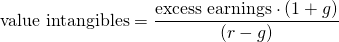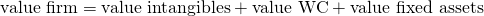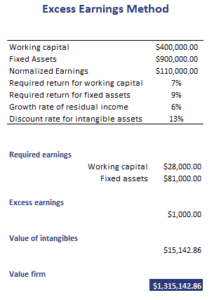# Excess Earnings Method

The Excess Earnings Method (EEM) is a method to value private firms. Under the EEM, the analyst starts with the earnings that should be generated by the working capital and the fixed assets based on the required rate of return. The excess earnings method is used when a private company has considerable amounts of intangible assets. The excess earnings method is comparable to residual income method. The main disadvantage of the approach is that is sensitive to estimation error.

On this page, we define excess earnings, discuss the excess earnings valuation method formula, and apply a numerical example using a spreadsheet. The spreadsheet is available for download at the bottom of the page.

# Excess earnings valuation method formula

Before we start off, we need to define excess earnings. Excess earnings equal the firm’s earnings minus the earnings that are required to provide the required rate of return on working capital (WC) and fixed assets. The value of the intangible assets can be estimated as the present value of a growing stream of excess earnings. The value of the intangible assets is then added to the value of working capital and fixed assets to determine the firm’s value.Once we have capitalized the excess earnings, the value of the firm equals:# Excess earnings method example

To get a better understanding of the different steps involved, let’s consider an example. The following table applies the above formulas to calculate the value of a firm with considerable intangible assets.The example clearly illustrates that the approach is very similar (actually, equivalent) to the single stage residual income model.

# Summary

We discussed the excess earnings valuation method. While the approach is not often used by analysts, it can nevertheless be useful to value small private companies with considerable intangible assets. The drawback of the approach is that it is sensitive to estimation error because value of the working capital and fixed assets have to be estimated.

### EEM calculator

Want to have an implementation in Excel? Download the Excel file: Excess Earnings calculator